# 1.3 Add and subtract integers  (Page 4/10)

 Page 4 / 10

In each case we got 8—either 8 positives or 8 negatives.

When the signs were the same, the counters were all the same color, and so we added them.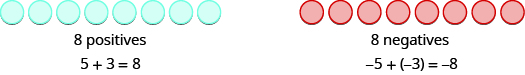Add: $1+4$ $-1+\left(-4\right).$

## Solution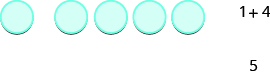1 positive plus 4 positives is 5 positives.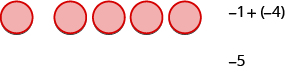1 negative plus 4 negatives is 5 negatives.

Add: $2+4$ $-2+\left(-4\right).$

6 $-6$

Add: $2+5$ $-2+\left(-5\right).$

7 $-7$

So what happens when the signs are different? Let’s add $-5+3.$ We realize this means the sum of $-5$ and 3. When the counters were the same color, we put them in a row. When the counters are a different color, we line them up under each other.

 −5 + 3 means the sum of −5 and 3. We start with 5 negatives.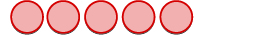And then we add 3 positives.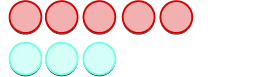We remove any neutral pairs.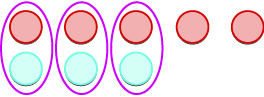We have 2 negatives left.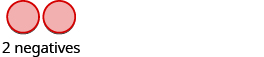The sum of −5 and 3 is −2. −5 + 3 = −2

Notice that there were more negatives than positives, so the result was negative.

Let’s now add the last combination, $5+\left(\text{−}3\right).$

 5 + (−3) means the sum of 5 and −3. We start with 5 positives.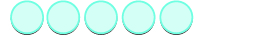And then we add 3 negatives.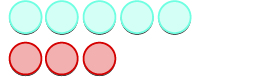We remove any neutral pairs.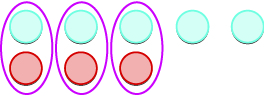We have 2 positives left.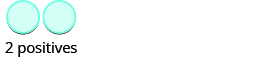The sum of 5 and −3 is 2. 5 + (−3) = 2

When we use counters to model addition of positive and negative integers, it is easy to see whether there are more positive or more negative counters. So we know whether the sum will be positive or negative.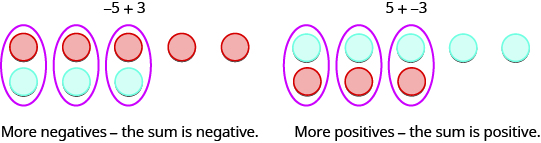Add: $-1+5$ $1+\left(-5\right).$

## Solution

 −1 + 5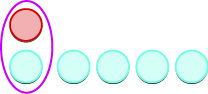There are more positives, so the sum is positive. 4

 1 + (−5)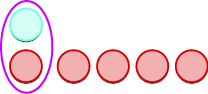There are more negatives, so the sum is negative. −4

Add: $-2+4$ $2+\left(-4\right).$

2 $-2$

Add: $-2+5$ $2+\left(-5\right).$

3 $-3$

Now that we have added small positive and negative integers with a model, we can visualize the model in our minds to simplify problems with any numbers.

When you need to add numbers such as $37+\left(\text{−}53\right),$ you really don’t want to have to count out 37 blue counters and 53 red counters. With the model in your mind, can you visualize what you would do to solve the problem?

Picture 37 blue counters with 53 red counters lined up underneath. Since there would be more red (negative) counters than blue (positive) counters, the sum would be negative . How many more red counters would there be? Because $53-37=16,$ there are 16 more red counters.

Therefore, the sum of $37+\left(\text{−}53\right)$ is $-16.$

$37+\left(\text{−}53\right)=-16$

Let’s try another one. We’ll add $-74+\left(\text{−}27\right).$ Again, imagine 74 red counters and 27 more red counters, so we’d have 101 red counters. This means the sum is $-101.$

$-74+\left(\text{−}27\right)=-101$

Let’s look again at the results of adding the different combinations of $5,-5$ and $3,-3.$

## Addition of positive and negative integers

$\begin{array}{cccc}\hfill 5+3\hfill & & & \hfill -5+\left(-3\right)\hfill \\ \hfill 8\hfill & & & \hfill -8\hfill \\ \hfill \text{both positive, sum positive}\hfill & & & \hfill \text{both negative, sum negative}\hfill \end{array}$

When the signs are the same, the counters would be all the same color, so add them.

$\begin{array}{cccc}\hfill -5+3\hfill & & & \hfill 5+\left(-3\right)\hfill \\ \hfill -2\hfill & & & \hfill 2\hfill \\ \hfill \text{different signs, more negatives, sum negative}\hfill & & & \hfill \text{different signs, more positives, sum positive}\hfill \end{array}$

When the signs are different, some of the counters would make neutral pairs, so subtract to see how many are left.

Visualize the model as you simplify the expressions in the following examples.

Simplify: $19+\left(-47\right)$ $-14+\left(-36\right).$

1. Since the signs are different, we subtract $\text{19 from 47}\text{.}$ The answer will be negative because there are more negatives than positives.
$\begin{array}{cccc}& & & \hfill \phantom{\rule{0.3em}{0ex}}19+\left(-47\right)\hfill \\ \text{Add.}\hfill & & & \hfill \phantom{\rule{0.3em}{0ex}}-28\hfill \end{array}$
2. Since the signs are the same, we add. The answer will be negative because there are only negatives.
$\begin{array}{cccc}& & & \hfill -14+\left(-36\right)\hfill \\ \text{Add.}\hfill & & & \hfill -50\hfill \end{array}$

Bruce drives his car for his job. The equation R=0.575m+42 models the relation between the amount in dollars, R, that he is reimbursed and the number of miles, m, he drives in one day. Find the amount Bruce is reimbursed on a day when he drives 220 miles.
LeBron needs 150 milliliters of a 30% solution of sulfuric acid for a lab experiment but only has access to a 25% and a 50% solution. How much of the 25% and how much of the 50% solution should he mix to make the 30% solution?
5%
Michael
hey everyone how to do algebra
Felecia answer 1.5 hours before he reaches her
I would like to solve the problem -6/2x
12x
Andrew
how
Christian
Does the x represent a number or does it need to be graphed ?
latonya
-3/x
Venugopal
-3x is correct
Atul
Arnold invested $64,000, some at 5.5% interest and the rest at 9%. How much did he invest at each rate if he received$4,500 in interest in one year?
Tickets for the community fair cost $12 for adults and$5 for children. On the first day of the fair, 312 tickets were sold for a total of $2204. How many adult tickets and how many child tickets were sold? Alpha Reply 220 gayla Three-fourths of the people at a concert are children. If there are 87 children, what is the total number of people at the concert? Tsimmuaj Reply Erica earned a total of$50,450 last year from her two jobs. The amount she earned from her job at the store was $1,250 more than four times the amount she earned from her job at the college. How much did she earn from her job at the college? Tsimmuaj Erica earned a total of$50,450 last year from her two jobs. The amount she earned from her job at the store was $1,250 more than four times the amount she earned from her job at the college. How much did she earn from her job at the college? Tsimmuaj ? Is there anything wrong with this passage I found the total sum for 2 jobs, but found why elaborate on extra If I total one week from the store *4 would = the month than the total is = x than x can't calculate 10 month of a year candido what would be wong candido 87 divided by 3 then multiply that by 4. 116 people total. Melissa the actual number that has 3 out of 4 of a whole pie candido was having a hard time finding Teddy use Matrices for the 2nd question Daniel One number is 11 less than the other number. If their sum is increased by 8, the result is 71. Find the numbers. Tsimmuaj Reply 26 + 37 = 63 + 8 = 71 gayla 26+37=63+8=71 ziad 11+52=63+8=71 Thisha how do we know the answer is correct? Thisha 23 is 11 less than 37. 23+37=63. 63+8=71. that is what the question asked for. gayla 23 +11 = 37. 23+37=63 63+8=71 Gayla by following the question. one number is 11 less than the other number 26+11=37 so 26+37=63+8=71 Gayla your answer did not fit the guidelines of the question 11 is 41 less than 52. gayla 71-8-11 =52 is this correct? Ruel let the number is 'x' and the other number is "x-11". if their sum is increased means: x+(x-11)+8 result will be 71. so x+(x-11)+8=71 2x-11+8=71 2x-3=71 2x=71+3 2x=74 1/2(2x=74)1/2 x=37 final answer tesfu just new Muwanga Amara currently sells televisions for company A at a salary of$17,000 plus a $100 commission for each television she sells. Company B offers her a position with a salary of$29,000 plus a $20 commission for each television she sells. How televisions would Amara need to sell for the options to be equal? Tsimmuaj Reply yes math Kenneth company A 13 company b 5. A 17,000+13×100=29,100 B 29,000+5×20=29,100 gayla need help with math to do tsi test Toocute me too Christian have you tried the TSI practice test ***tsipracticetest.com gayla DaMarcus and Fabian live 23 miles apart and play soccer at a park between their homes. DaMarcus rode his bike for 34 of an hour and Fabian rode his bike for 12 of an hour to get to the park. Fabian’s speed was 6 miles per hour faster than DaMarcus’s speed. Find the speed of both soccer players. gustavo Reply ? Ann DaMarcus: 16 mi/hr Fabian: 22 mi/hr Sherman Joy is preparing 20 liters of a 25% saline solution. She has only a 40% solution and a 10% solution in her lab. How many liters of the 40% solution and how many liters of the 10% solution should she mix to make the 25% solution? Wenda Reply 15 and 5 32 is 40% , & 8 is 10 % , & any 4 letters is 5%. Karen It felt that something is missing on the question like: 40% of what solution? 10% of what solution? Jhea its confusing Sparcast 3% & 2% to complete the 25% Sparcast because she already has 20 liters. Sparcast ok I was a little confused I agree 15% & 5% Sparcast 8,2 Karen Jim and Debbie earned$7200. Debbie earned \$1600 more than Jim earned. How much did they earned
5600
Gloria
1600
Gloria
Bebbie: 4,400 Jim: 2,800
Jhea
A river cruise boat sailed 80 miles down the Mississippi River for 4 hours. It took 5 hours to return. Find the rate of the cruise boat in still water and the rate of the current.
A veterinarian is enclosing a rectangular outdoor running area against his building for the dogs he cares for. He needs to maximize the area using 100 feet of fencing. The quadratic equation A=x(100−2x) gives the area, A , of the dog run for the length, x , of the building that will border the dog run. Find the length of the building that should border the dog run to give the maximum area, and then find the maximum area of the dog run.
ggfcc
MikeByBy Nick SwainByByBy Mldelatte